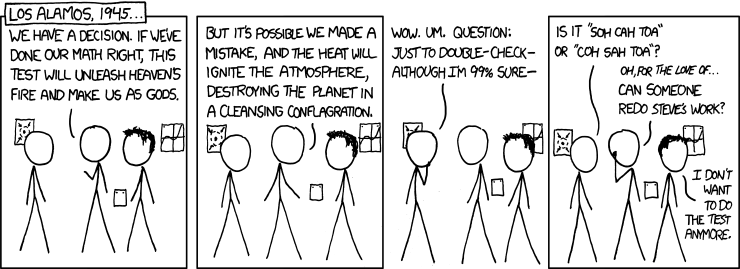# Calculus - Trigonometry, differentiation.

• Posted on: 28 January 2021
Block:
Term:
Year:
LA Code:
Code:
Room:
Learning Area:
Levels:
8

This is normally a full year course for students who have completed level two mathematics. It will prepare students for first year university courses in mathematics, computer science, physics and engineering as well as providing a foundation for a future studies in subjects such as biomathematics and economics.The ncea year plan is as follows:

• Trigonometry - internal assessment 91575 4cr, test date around week 6
• Differentiation - external assessment 91578 6cr
• Integration - external assessment 91579 6cr
• Complex Numbers - external assessment 91577 5cr

I suggest all students revise basic algebra as it is the language we will be using in this course to learn more complex ideas. Maybe create a khan academy login and practise - you can join the rest of the class on there with this code 5E2XNC
These are the two key areas to be well practised at.

Apply trigonometric methods in solving problems
In the trigonometry unit we introduce two important ideas, proofs and modelling.
The internal for trigonometry is completed online using the desmos graphing tool. It should take about 5 weeks to cover the content for this topic then we will move onto differentiation.

Achievement criteria

Achievement
You need to apply trigonometric methods in solving problems
This could involve:

• Selecting and using methods.
• demonstrating knowledge of concepts and terms.
• communicating using appropriate representations.
• Problems are situations that provide opportunities to apply knowledge or understanding of mathematical concepts and methods. Situations will be set in a real-life or mathematical contexts.

You need to be familiar with:

• trigonometric identities.
• reciprocal trigonometric functions.
• features of trigonometric functions.
• solving trigonometric equations.
• general solutions.

Achievement with Merit
Make sure that you can meet the criteria for achievement
You need to apply trigonometric methods, using relational thinking, in solving problems.
This could involve one or more of:

• carrying out a logical sequence of steps.
• connecting different concepts or representations.
• demonstrating understanding of concepts.
• forming and using a model.
• and also relating findings to a context, or communicating thinking using appropriate mathematical statements.

Achievement with Excellence
Make sure that you can meet the criteria for merit
You need to apply trigonometric methods, using extended abstract thinking, in solving problems.
This could involve one or more of:

• devising a strategy to investigate or solve a problem.
• identifying relevant concepts in context.
• developing a chain of logical reasoning, or proof.
• forming a generalisation.
• and also using correct mathematical statements, or communicating mathematical insight.

Assessment Opportunities: